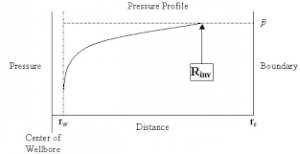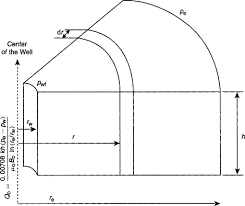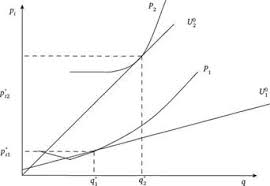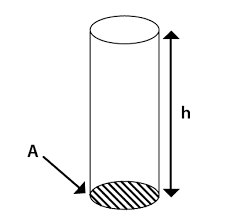## How to Calculate and Solve for Reservoir Radius and Well Spacing | The Calculator EncyclopediaThe image above represents the reservoir radius.

To compute for the reservoir radius, one essential parameter is needed and this parameter is Well Spacing (A).

The formula for calculating the reservoir radius:

re = √[43560A  / π]

Where;

A = Well Spacing

Let’s solve an example;
Given that the well spacing of an reservoir radius is 42. Find the reservoir radius?

This implies that;

A = Well Spacing = 42

re = √[43560A  / π]
re = √[43560 x 42 / π]
re = √[1829520 / π]
re = √582354.3
re = 763.12

Therefore, the reservoir radius is 763.12 ft.

## How to Calculate and Solve for Radial Flow Rate in Reservoir Fluid Flow | The Calculator EncyclopediaThe image above represents the radial flow rate.

To compute for the radial flow rate, eight essential parameters are needed and these parameters are External Pressure (Pe), Flowing Bottom-Hole Pressure (Pwf), Formation Thickness (h), Oil Viscosity (μo ), Permeability (k), Oil Formation Volume Factor (Bo), Drainage Radius (reand Well Bore Radius (rw ).

The formula for calculating the radial flow rate:

Qo = 0.00708kh[Pe – Pwf] / μo Bo In[re / rw]

Where;

Pe = External Pressure
Pwf = Flowing Bottom-Hole Pressure
h = Formation Thickness
μo = Oil Viscosity
k = Permeability
Bo = Oil Formation Volume Factor

Let’s solve an example;
Find the radial flow rate when the External Pressure is 14, Flowing Bottom-Hole Pressure is 21, Formation Thickness is 7, Oil Viscosity is 35, Permeability is 50, Oil Formation Volume Factor is 13, Drainage Radius is 26 and Well Bore Radius is 15.

This implies that;

Pe = External Pressure = 14
Pwf = Flowing Bottom-Hole Pressure = 21
h = Formation Thickness = 7
μo = Oil Viscosity = 35
k = Permeability = 50
Bo = Oil Formation Volume Factor = 13
re = Drainage Radius = 26
rw = Well Bore Radius = 15

Qo = 0.00708kh[Pe – Pwf] / μo Bo In[re / rw]
Qo = 0.00708 x 50 x 7 [14 – 21] / 35 x 13 In[26 / 15]
Qo = 0.00708 x 50 x 7 [-7] / 35 x 13 In[26 / 15]
Qo = 0.00708 x 50 x 7 [-7] / 35 x 13 In[1.73]
Qo = 0.00708 x 50 x 7 [-7] /35 x 13 x 0.55
Qo = 2.478 [-7] / 35 x 13 x 0.55
Qo = -17.346 / 250.27
Qo = -0.069

Therefore, the radial flow rate is -0.069 STB/day.

## How to Calculate and Solve for Linear Flow Rate in Reservoir Fluid Flow | The Calculator EncyclopediaThe image above represents the linear flow rate.

To compute for the linear flow rate, four parameters are needed and these parameters are Initial pressure (P1), Final Pressure (P2), Thickness (h) and Permeability (k).

The formula for calculating linear flow rate:

q = 0.001127kh[P1 – P2]

Where:
q = Linear Flow Rate
P1 = Initial Pressure
P2 = Final Pressure
h = Thickness
k = Permeability

Let’s solve an example;
Find the linear flow rate when the initial pressure is 12, final pressure is 22, thickness is 18 and permeability is 44.

This implies that;
P1 = Initial Pressure = 12
P2 = Final Pressure = 22
h = Thickness = 18
k = Permeability = 44

q = 0.001127kh [P1 – P2]
q = 0.001127 x 44 x 18 [12 – 22]
q = 0.001127 x 44 x 18 [-10]
q = 0.892584 [-10]
q = -8.92584

Therefore, the linear flow rate is -8.92584 bbl/day.

Calculating Permeability when the linear flow rate, Initial Pressure, Final Pressure and Thickness is Given.

k = q / 0.001127h (p1 – p2)

Where;
k = Permeability
q = Linear Flow Rate
P1 = Initial Pressure
P2 = Final Pressure
h = Thickness

Let’s solve an example;
Find the permeability when the initial pressure is 34, final pressure is 24, thickness is 12 and linear flow rate is 50.

This implies that;
q = Linear Flow Rate = 50
P1 = Initial Pressure = 34
P2 = Final Pressure = 24
h = Thickness = 12

k = q / 0.001127h (p1 – p2)
k = 50 / 0.001127h (34 – 24)
k = 50 / 0.001127h (10)
k = 50 / 0.01127
k = 4436.5

Therefore, the permeability is 4436.5.

## How to Calculate and Solve for Fluid Potential, Pressure, Datum Level and Density | The Calculator EncyclopediaThe image above represents the fluid potential.

To compute for the fluid potential, three essential parameters are needed and these parameters are pressure (P), Datum Levels (ΔZ) and Density (ρ).

The formula for calculating fluid potential:

φ = P – [ρ / 144]ΔZ

Where;
φ = Fluid Potential
P = Pressure
ΔZ = Datum Levels
ρ = Density

Let’s solve an example;
Find the fluid potential when the pressure is 24 with a datum level of 18 and the density of 30.

This implies that;
P = Pressure = 24
ΔZ = Datum Levels = 18
ρ = Density = 30

φ = P – [ρ / 144]ΔZ
φ = 24 – [30/144] 18
φ = 24 – [0.2083] 18
φ = 24 – 3.75
φ = 20.25

Therefore, the fluid potential is 20.25.

Calculating the Pressure(P) when the fluid potential, Datum levels and Density is Given.

P = φ – [ρ / 144]ΔZ

Where;
P = Pressure
φ = Fluid Potential
ΔZ = Datum Levels
ρ = Density

Lets solve an example;
Find the pressure with a fluid potential of 40 and a datum levels of 18 with density of 24.

This implies that;
φ = Fluid Potential = 40
ΔZ = Datum Levels = 18
ρ = Density = 24

P = φ – [ρ / 144]ΔZ
P = 40 – [24 / 144]18
P = 40 – [0.167]18
P = 40 – 3
P = 37

Therefore, the pressure is 37.